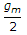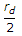# Electronics and Communication Engineering - Analog Electronics

26.

Two identical FETs, each characterised by the parameters gm and rd are connected in parallel. The composite FET is then characterised by the parameters

 A.and 2rd B.andC. 2gm andD. 2gm and 2rd

Explanation:

No answer description available for this question. Let us discuss.

27.

Assertion (A): If power gain doubles the power gain increases by 3 dB

Reason (R): The bel power gain of cascaded stages is equal to sum of each bel power gain

 A. Both A and R are correct and R is correct explanation for A B. Both A and R are correct but R is not correct explanation for A C. A is correct R is wrong D. A is wrong R is correct

Explanation:

No answer description available for this question. Let us discuss.

28.

The V MOS can handle __________ currents than a conventional MOSFET.

 A. much lower B. almost same C. almost half D. much larger

Explanation:

No answer description available for this question. Let us discuss.

29.

Assertion (A): A resistor is a passive load and a transistor is an active load

Reason (R): Op-amp is mostly used in feedback mode

 A. Both A and R are correct and R is correct explanation for A B. Both A and R are correct but R is not correct explanation for A C. A is correct R is wrong D. A is wrong R is correct

Explanation:

No answer description available for this question. Let us discuss.

30.

Which mode is usually preferred for cascading?

 A. CE B. CB C. CC D. Any of the above